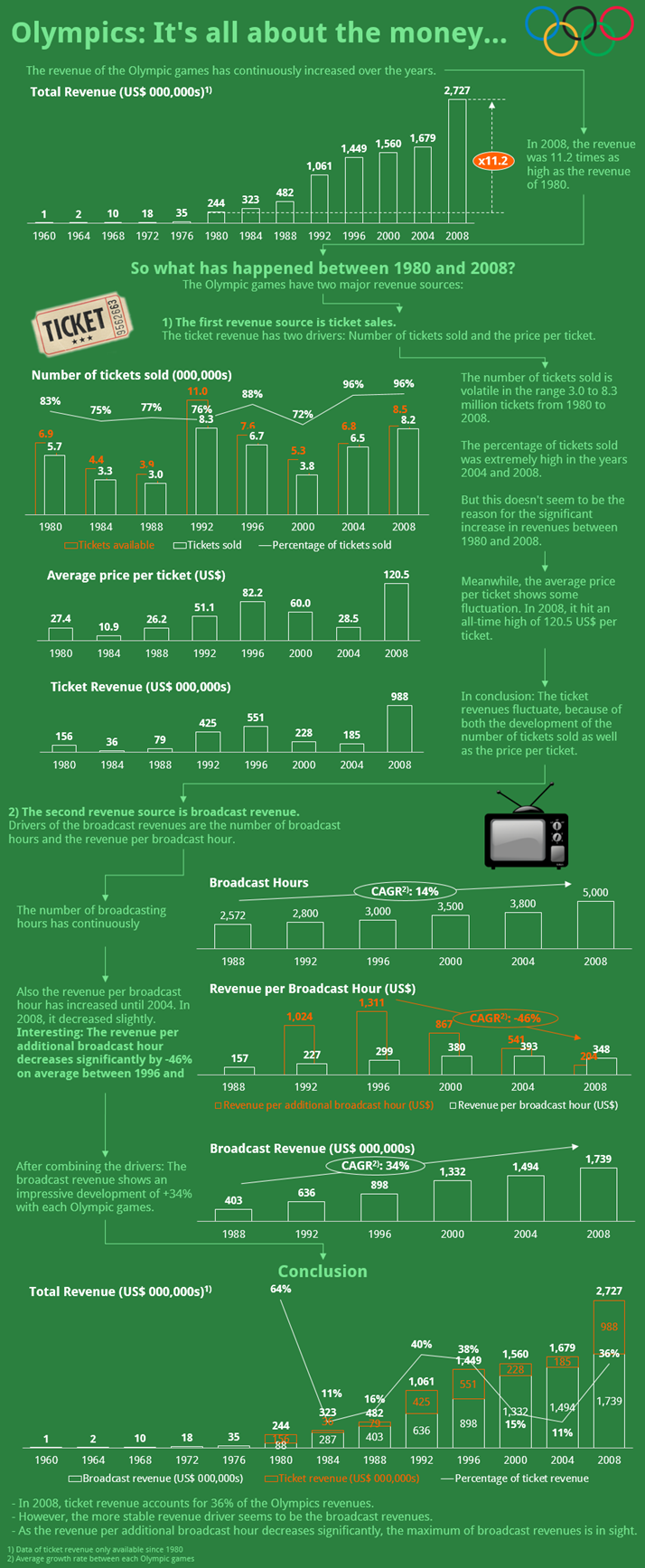#### IFERROR: How to Handle Error Messages in Excel

When your formula produces an error in Excel – for example #N/A or #VALUE? – you got two options: Solve the error or use it in your calculation. Solving is usually a good idea but not always possible. So let’s take a look at how to deal with errors in Excel formulas using the IFERROR formula.

#### Fun in Excel: 5 Ways of Fun with SpreadsheetsLet’s have some fun in Excel! You probably do your work with spreadsheets, but there are also ways of having fun in Excel. In this article, we introduce you to 5 fun applications for Microsoft Excel.

Sometimes Excel workbooks become quite large: The more worksheets there are, the more difficult it is to keep the overview. A table of contents might help. In this article we’ll explore 4 ways of creating tables of contents in an Excel workbook.

#### Copy Exact Formulas: 4 Ways of Preserving the Cell Links in ExcelThis problem occurs quite often: Excel always adapts the cell ranges in formulas when copying cells. An example: Cell A1 links to A2. When you copy A1 to B1, the link will change to B2. This example is comparatively simple but there are more complex situations. In this article, we’ll take a look at four methods for copying and pasting the exact formulas in Excel: Two manual ways by using the \$-sign or copying the cell contents, the third method by using a simple replace trick and the fourth: the automatic method.

#### Sum in Excel: 8 Ways Of Adding Up Values

One of the basic applications in Excel is summing up values. The most popular ways of adding up numbers are just using the ‘+’ sign or the formula SUM. But there are many other methods. Do you know about these 6 other ways to sum up values in Excel? In this article we’ll take a look at 8 methods for summing up values in Excel and compare them.

#### Avoid Errors in Excel: 6 Strategies to Prevent Mistakes

In our last article, we have seen the 5 biggest fails in Excel. But instead of laughing about these mistake, we should rather talk about how to find and avoid errors. In this article, we’re taking a look at 6 methods of how to prevent errors in your spreadsheet.

#### INDEX and MATCH: The Alternative to VLOOKUP in Excel

You’ve probably heard of the VLOOKUP formula in Excel, haven’t you? The VLOOKUP formula searches for a value in a column. Once found it returns another value from the same row. A combination of INDEX and MATCH serves the same purpose. It works slightly different and has therefore some advantages and disadvantages towards VLOOKUP.

#### Break Links in Excel – All of Them (Even When Excel Doesn’t)

When you copy cells or worksheets from another Excel workbook, links to other worksheets in many cases still persists. Excel offers a function to break links but this function only works with links within formulas. There are many other types of links as links within conditional formatting rules or data validation rules. The bad news: Those links can’t be cut easily. The good news: there are still ways to break these links.

#### 7 Simple Tricks to Make An Excel Workbook Look Professional

Creating Excel workbooks is often a long process: Setting up the structure, importing inputs, conducting the calculations and eventually tidying it up and sharing it. So once you are done organizing the contents, you have to make sure that the contents are delivered and received well. Therefore it’s crucial that the workbook shows a certain level of professionalism. In this article, we’ll explore 7 simple tricks for making your Excel workbook look professional.

#### Excel World Champ – Round 2 – Henrik Schiffner